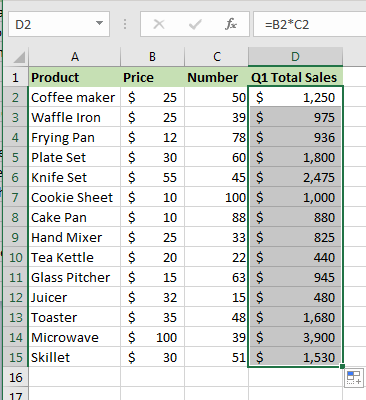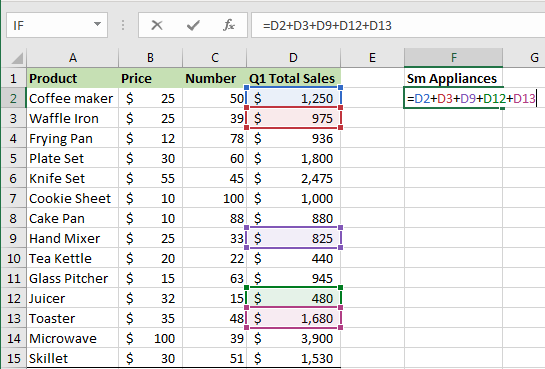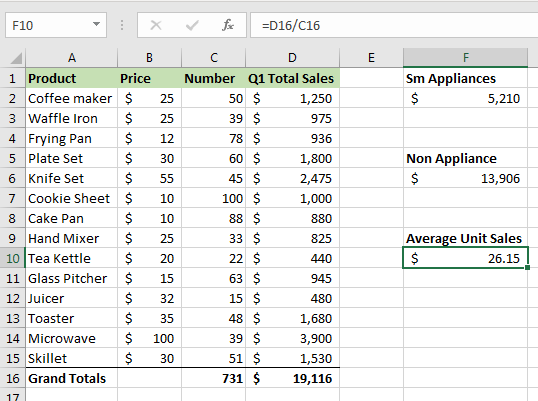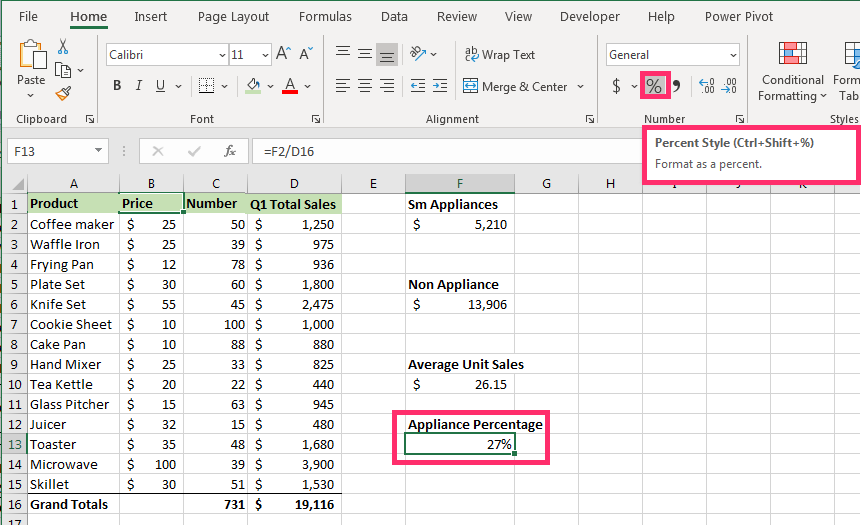# 4.21: Simple Math

$$\newcommand{\vecs}{\overset { \rightharpoonup} {\mathbf{#1}} }$$ $$\newcommand{\vecd}{\overset{-\!-\!\rightharpoonup}{\vphantom{a}\smash {#1}}}$$$$\newcommand{\id}{\mathrm{id}}$$ $$\newcommand{\Span}{\mathrm{span}}$$ $$\newcommand{\kernel}{\mathrm{null}\,}$$ $$\newcommand{\range}{\mathrm{range}\,}$$ $$\newcommand{\RealPart}{\mathrm{Re}}$$ $$\newcommand{\ImaginaryPart}{\mathrm{Im}}$$ $$\newcommand{\Argument}{\mathrm{Arg}}$$ $$\newcommand{\norm}{\| #1 \|}$$ $$\newcommand{\inner}{\langle #1, #2 \rangle}$$ $$\newcommand{\Span}{\mathrm{span}}$$ $$\newcommand{\id}{\mathrm{id}}$$ $$\newcommand{\Span}{\mathrm{span}}$$ $$\newcommand{\kernel}{\mathrm{null}\,}$$ $$\newcommand{\range}{\mathrm{range}\,}$$ $$\newcommand{\RealPart}{\mathrm{Re}}$$ $$\newcommand{\ImaginaryPart}{\mathrm{Im}}$$ $$\newcommand{\Argument}{\mathrm{Arg}}$$ $$\newcommand{\norm}{\| #1 \|}$$ $$\newcommand{\inner}{\langle #1, #2 \rangle}$$ $$\newcommand{\Span}{\mathrm{span}}$$$$\newcommand{\AA}{\unicode[.8,0]{x212B}}$$

Learning Objectives

• Complete simple math functions in Excel

Excel spreadsheets are often used for calculating numbers with simple math. There are a few things to learn to set up using simple math calculations in Excel. You’ve already learned about the SUM function. This next section will teach other simple math steps to use in spreadsheets.

The basic math calculation steps in Excel are set up with the background of first quarter sales numbers for a department store. The example table includes the product type, sales price, number of items sold and the total for each item. Start by figuring out the total sales for each product type for the quarter.

## Multiplication

• Total sales for each product requires the multiplication of sales price and number sold.
• Select the cell in Q1 total sales column and type in = Price Cell * Number Cell.
• Press the keyboard Enter key and the total is calculated.
• Type the same formula in for each cell or use the flash fill way by dragging the bottom right corner down the entire column and it will fill with the total sales for each product.• To find total sales for just small appliances, add their sales totals together by using the plus sign.
• Select the cell under total the Sm Appliances and type in = Q1 Total Sales Cell Reference for Product1 + Q1 Total Sales Cell Reference for Product2 + Q1 Total Sales Cell Reference for Product3 + Q1 Total Sales Cell Reference for Product4 , etc. (for this example, the Microwave is not a small appliance).
• Press the keyboard Enter key.
• Total sales for all small appliance sales is displayed.
• Another way to write this addition formula is by using brackets =SUM(D2+D3+D9+D12+D13).## Subtraction

• For the total sales for all products, except small appliances, subtract the grand total from the small appliance total using the subtraction sign.
• Select the cell under total the Non-Appliance and type in = Grand Totals Sales Cell Reference – Sm Appliance total.
• Press the keyboard Enter key.
• Another way to write this subtraction formula is =SUM(D16-F2).## Division

• Maybe you need to know what the average unit sale would be across all products for the first quarter. In that case, divide the grand total sales by the number of units sold using the division sign
• Select the cell under Average Unit Sales title and type in = Grand Totals Sales Cell Reference / Number.
• Press the keyboard Enter key.
• You will now see what the average product sale was for Q1.
• Another way to write this division/average formula is =SUM(D16/C16).## Percentage

• To find what the percentage of sales for small appliances out of total sales was, divide the total small appliance sales by the grand total sales using the division sign.
• Select the cell under Appliance Percentage title and type in = Sm Appliances / Grand Totals Sales Cell Reference.
• Press the keyboard Enter key.
• The average product sale was for Q1 displays as a decimal point.
• Another way to write this percentage formula is =SUM(F2/D16).To have the results display as a percentage, click the % button on the Home tab in the Number group.Note

More than one calculation can be included in a single cell’s formula. For example, you want to know the total sales of a few specific items for Q1; coffee maker, tea kettle and microwave.

If the Q1 totals hadn’t been calculated yet, you’d need to multiply the number and sales price of each item then add up the total. This can be written in one formula by placing a comma between calculations. It is calculated like this =SUM(B2*C2,B10*C10,B14*C14).